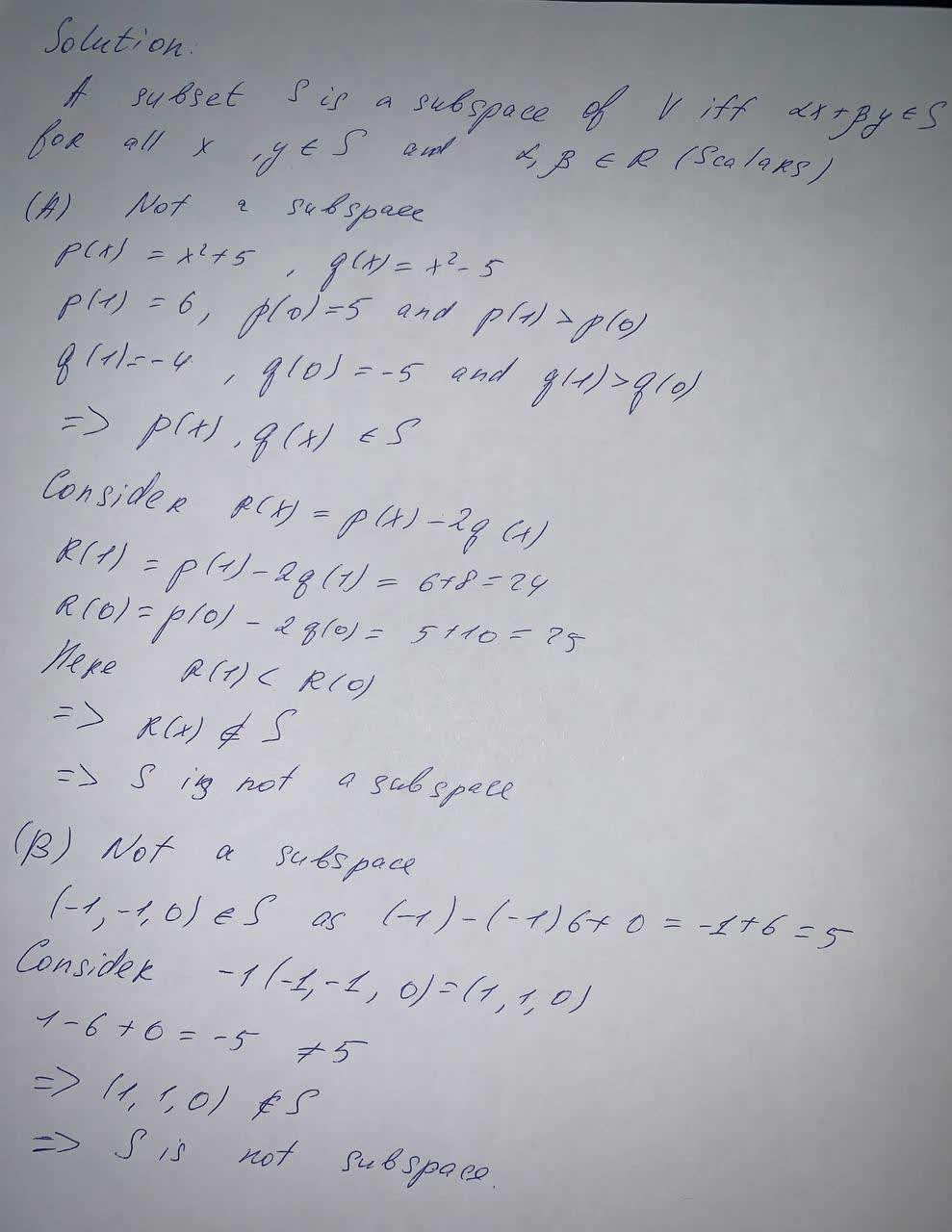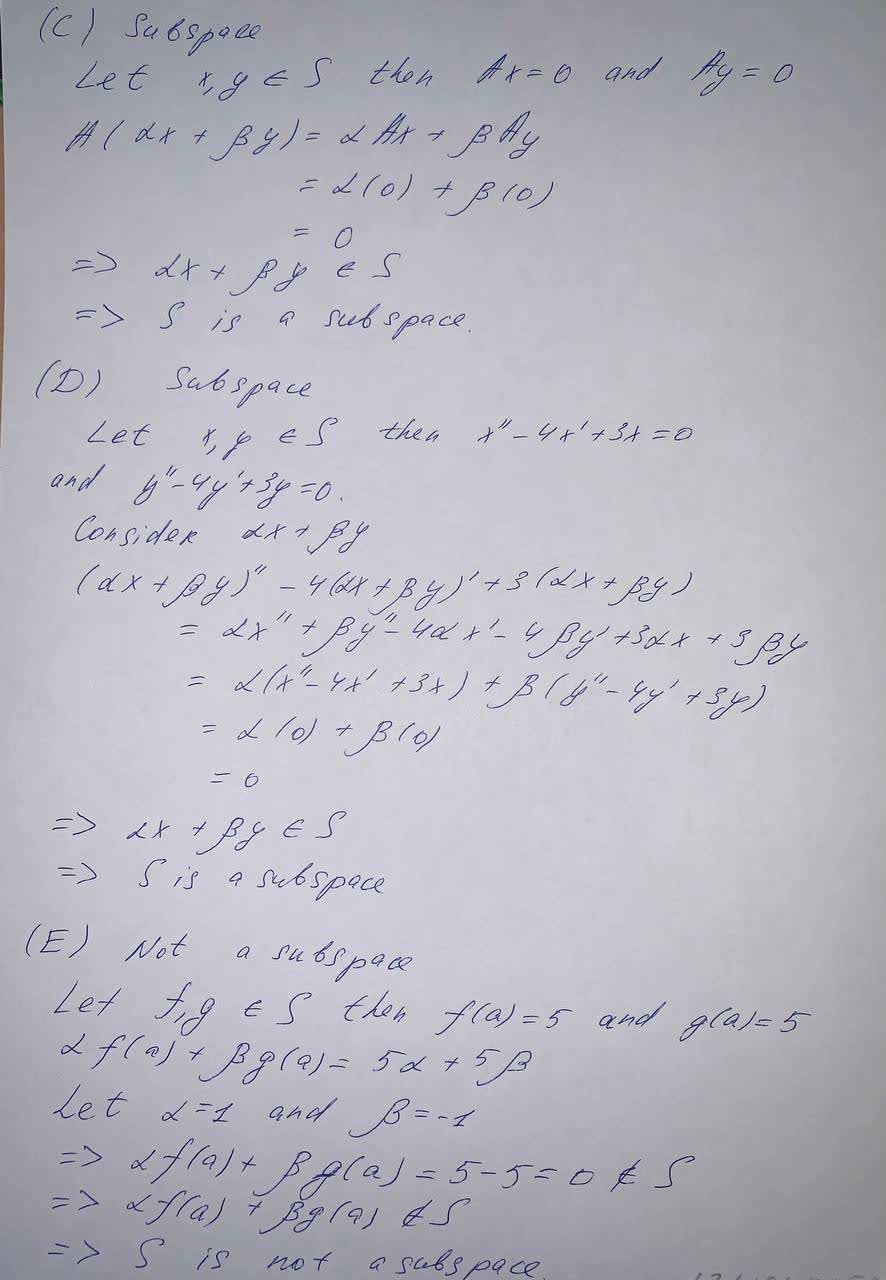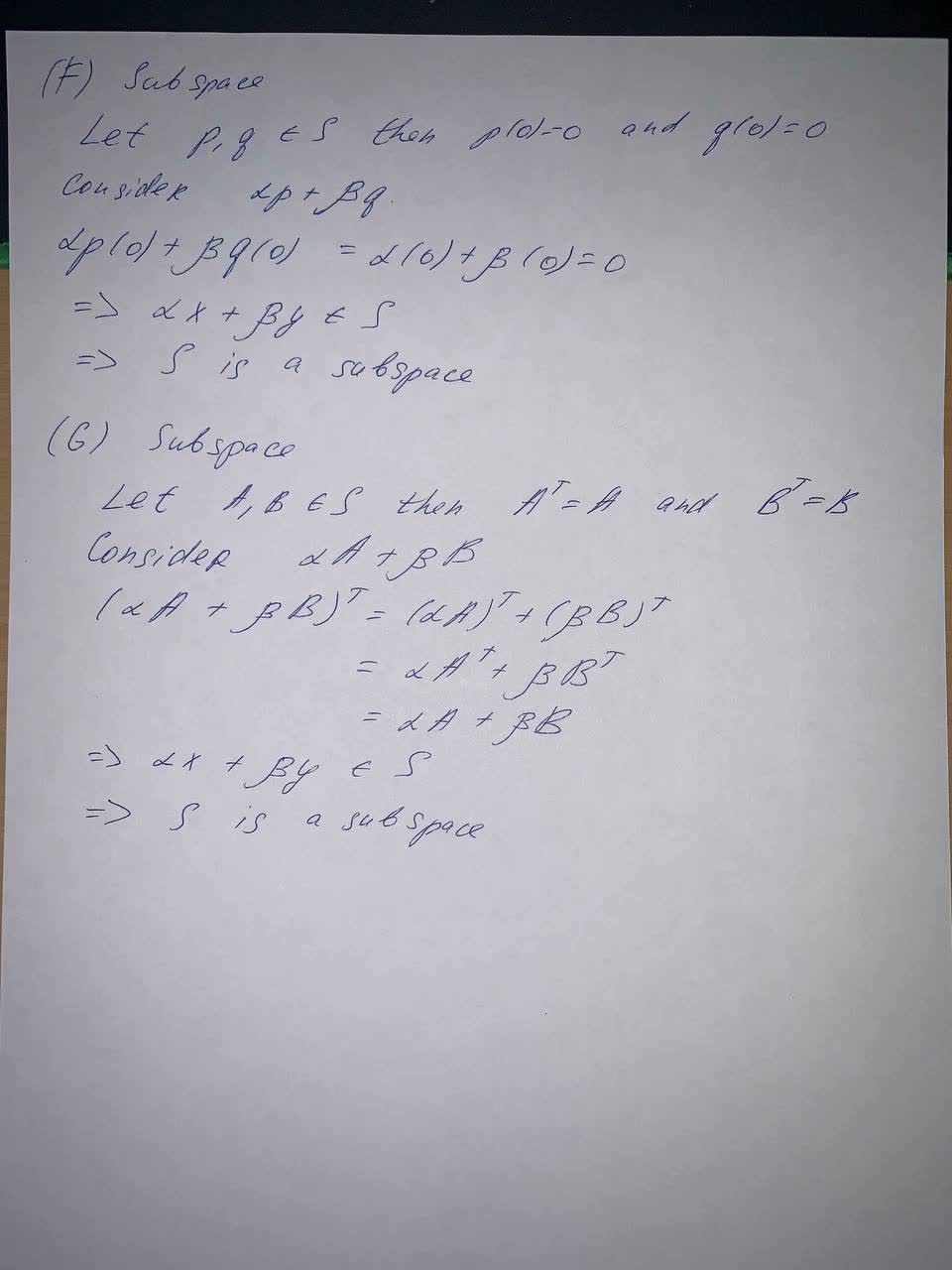#### Didn’t find what you are looking for?

Question# Determine whether the given set S is a subspace of the vector space V. A. V=P_5, and S is the subset of P_5 consisting of those polynomials satisfying

Forms of linear equations
ANSWEREDDetermine whether the given set S is a subspace of the vector space V.
A. V=$$P_5$$, and S is the subset of $$P_5$$ consisting of those polynomials satisfying p(1)>p(0).
B. $$V=R_3$$, and S is the set of vectors $$(x_1,x_2,x_3)$$ in V satisfying $$x_1-6x_2+x_3=5$$.
C. $$V=R^n$$, and S is the set of solutions to the homogeneous linear system Ax=0 where A is a fixed m×n matrix.
D. V=$$C^2(I)$$, and S is the subset of V consisting of those functions satisfying the differential equation y″−4y′+3y=0.
E. V is the vector space of all real-valued functions defined on the interval [a,b], and S is the subset of V consisting of those functions satisfying f(a)=5.
F. V=$$P_n$$, and S is the subset of $$P_n$$ consisting of those polynomials satisfying p(0)=0.
G. $$V=M_n(R)$$, and S is the subset of all symmetric matrices